## Study Questions

— With QuizzerWeb

##### MATHEMATICS QUIZ STUDY
The Correct Options are highlighted thus - >
Correct Answer
.

Please share this quiz link to your friends, they might need it! Click here to Practice MATHEMATICS in CBT mode

1.

Convert 726 to a number in base three.

.
A.

2211

B.

2121

C.

1212

D.

1122

2.

Convert 2710 to another number in base three.

.
A.

10103

B.

11003

C.

10003

D.

10013

3.
3 girls share a number of apples in the ratio 5:3:2. If the highest share is 40 apples, find the smallest share.
.
A. 24
B. 16
C. 38
D. 36

4.

Evaluate , correct to 1 decimal place.

.
A.

6.2

B.

6.3

C.

0.5

D.

0.6

5.

Calculate the time taken for N3,000 to earn N600 if invested at 8% simple interest.

.
A.

3 years

B.

$3\frac{1}{2}$ years

C.

$1\frac{1}{2}$ years

D.

$2\frac{1}{2}$ years.

6.

Simplify

.
A.

33

B.

35

C.

3

D.

32

7.

If log 104 = 0.6021, evaluate log10 $4\frac{1}{2}$

.
A.

0.9021

B.

1.8063

C.

0.2007

D.

0.3011

8.

Simplify $\frac{\sqrt{5}\left(\sqrt{147}-\sqrt{12}\right)}{\sqrt{15}}$

.
A.

1/5

B.

1/9

C.

9

D.

5

9.

P, Q and R are subsets of the universal set U. The venn diagram showing the relationship (P $\cap$ Q) $\cup$ R is

.
A..

B.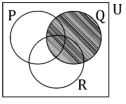.

C.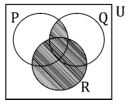.

D..

10.

If P = { x : x is odd, -1 < x ≤ 20 } and Q = { y : y is prime, -2 < y ≤ 25 }, find P $\cap$ Q

.
A.

{3,5,11,13,17,19}

B.

{3,5,7,11,13,17,19}

C.

{2,3,5,7,11,13,17,19}

D.

{3,5,7,11,17,19}

11.

If S = $\sqrt{{t}^{2}-4t+4}$, find t in terms of S.

.
A.

S + 2

B.

S – 2

C.

S2 + 2

D.

S2 – 2

12.

if x – 4 is a factor of x2 – x – k, then k is

.
A.

12

B.

20

C.

2

D.

4

13.

The remainder when 6p2 - p2 - 47p + 30 is divided by p – 3 is

.
A.

42

B.

63

C.

18

D.

21

14.

P varies jointly as m and u, and varies inversely as q. Given that p=4, m=3 and u= 2 when q = 1, find the value of p when m = 6, u = 4 and q =  3/5.

.
A.

15

B.

10

C.

200/5

D.

120/5

15.

If r varies inversely as the square root of s and t, how does s vary with r and t?

.
A.

s varies inversely as r2 and t.

B.

s varies directly as r2 and t2 .

C.

s varies directly as r and t.

D.

s varies inversely as r and t2 .

16.
Evaluate 3(x + 2) > 6(x + 3)
.
A. x > −4
B. x < −4
C. x > 4
D. x < 4

17.The graph above is correctly represented by

.
A.

y = x2 – 3x + 2

B.

y = x2 – x – 1

C.

y = x2 + x – 2

D.

y = x2 – x – 2

18.

Solve for x: [x - 2] < 3

.
A.

−2 < x < 3

B.

−1 < x < 5

C.

x < 1

D.

x < 5

19.
If the sum of the first two terms of a G. P is 3, and the sum of the second and the third terms is −6, find the sum of the first term and the common ratio.
.
A. −3
B. −5
C. 5
D. −2

20.

The nth term of the progression $\frac{4}{2},\frac{7}{3},\frac{10}{4},\frac{13}{5}$... is

.
A.

$\frac{3n+1}{n+1}$

B.

$\frac{3n+1}{n-1}$

C.

$\frac{3n-1}{n+1}$

D.

$\frac{1-3n}{n+1}$

21.

If a binary operation ∗ is defined by  , find 2 * (3 * 4)

.
A.

16

B.

14

C.

26

D.

24

22.

If  and , find 2P + Q.

.
A.

$\left[\begin{array}{cc}14& 8\\ 7& 7\end{array}\right]$

B.

$\left[\begin{array}{cc}7& 7\\ 8& 14\end{array}\right]$

C.

$\left[\begin{array}{cc}8& 14\\ 7& 7\end{array}\right]$

D.

$\left[\begin{array}{cc}7& 7\\ 14& 8\end{array}\right]$

23.

Find the inverse of $\left[\begin{array}{cc}5& 3\\ 6& 4\end{array}\right]$

.
A.

$\left[\begin{array}{cc}2& -\frac{3}{2}\\ -3& \frac{5}{2}\end{array}\right]$

B.

$\left[\begin{array}{cc}2& \frac{3}{2}\\ -3& -\frac{5}{2}\end{array}\right]$

C.

$\left[\begin{array}{cc}2& \frac{3}{2}\\ -3& \frac{5}{2}\end{array}\right]$

D.

$\left[\begin{array}{cc}2& -\frac{3}{2}\\ -3& -\frac{5}{2}\end{array}\right]$

24.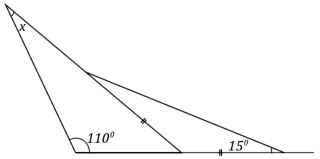In the diagram above, find the value of x.

.
A.

40º

B.

45º

C.

15º

D.

30º

25.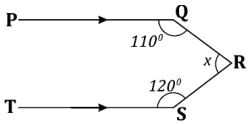The value of x in the figure above is

.
A.

100º

B.

70º

C.

130º

D.

110º

26.

If the angles of a quadrilateral are (3y + 10)º , (2y + 30)º , (y + 20)º and 4yº , find the value of y.

.
A.

42º

B.

66º

C.

12º

D.

30º

27.

A square tile has side 30cm. How many of these tiles will cover a rectangular floor of length 7.2m and width 4.2m?

.
A.

576

B.

720

C.

336

D.

420

28.

Find the length of a chord which subtends an angle 90º at the centre whose radius is 8cm.

.
A.

$8\sqrt{2}$cm

B.

$8\sqrt{3}$cm

C.

4 cm

D.

8 cm

29.

A chord of a circle subtends an angle 120º at the centre of a circle of diameter $4\sqrt{3}$cm. Calculate the area of the major sector.

.
A.

16π cm2

B.

32π cm2

C.

4π cm2

D.

8π cm2

30.
The locus of the points which is equidistant from the line PQ forms a
.
A. pair of parallel lines to PQ
B. perpendicular line to PQ
C. circle centre P
D. circle centre Q.

31.

If the mid-point of the line PQ is (2, 3) and the point P is (−2, 1), find the coordinate of the point Q.

.
A.

(6, 3)

B.

(8, 6)

C.

(5, 6)

D.

(0, 4)

32.
Find the equation of the perpendicular bisector of the line joining P(2, −3) to Q(−5, 1).
.
A. 8y + 14x – 13 = 0
B. 8y + 14x + 13 = 0
C. 8y – 14x + 13 = 0
D. 8y – 14x – 13 = 0

33.

In triangle PQR, q = 8 cm, r = 6 cm and cos P =$\frac{1}{12}$ . Calculate the value of p.

.
A.

10 cm

B.

$\sqrt{108}$cm

C.

9 cm

D.

$\sqrt{92}$cm

34.

If tanθ = $\frac{3}{4}$, find the value of sin θ + cos θ.

.
A.

$1\frac{2}{5}$

B.

$1\frac{1}{3}$

C.

$1\frac{2}{3}$

D.

$1\frac{3}{5}$

35.

If y = (2x + 2)3 , find $\frac{dy}{dx}$

.
A.

6(2x + 2)

B.

3(2x + 2)

C.

6(2x + 2)2

D.

3(2x + 2)2

36.

If y = x sin x, find $\frac{dy}{dx}$

.
A.

cos x – x sin x

B.

cos x + x sin x

C.

sin x + x sin x

D.

sin x – cos x.

37.

The radius of a circle is increasing at the rate of 0.02 cms-1 . Find the rate at which the area is increasing when the radius of the circle is 7cm.

.
A.

0.53 cm-2s-1

B.

0.35 cm-2s-1

C.

0.88 cm-2s-1

D.

0.75 cm-2s-1

38.

Integrate

.
A.

${x}^{2}-\frac{1}{x}+k$

B.

$2{x}^{2}-\frac{1}{x}+k$

C.

$-\frac{1}{2{x}^{2}}-\frac{1}{x}+k$

D.

$-\frac{{x}^{2}}{2}-\frac{1}{x}+k$

39.

Evaluate

.
A.

−1

B.

−2

C.

2

D.

1

40.The bar chart above shows the allotment of time (in minutes) per week for selected subjects in a certain school. What is the total time allocated to the six subjects per week?

.
A.

720 mins.

B.

960 mins.

C.

200 mins.

D.

460 mins.

41.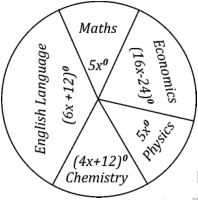The pie chart above shows the statistical distribution of 80 students in five subjects in an examination. Calculate how many students offer Mathematics.

.
A.

40

B.

50

C.

12

D.

30

42.
Find the mean of t + 2, 2t – 4, 3t + 2 and 2t.
.
A. 2t
B. 2t + 1
C. t
D. t + 1

43.
The mean of seven numbers is 10. If six of the numbers are 2, 4, 8, 14, 16 and 18, find the mode.
.
A. 8
B. 14
C. 2
D. 6

44.
 Age 20 25 30 35 40 45 No. of people 3 5 1 1 2 3

Calculate the median age of the frequency distribution in the table above.

.
A.

30

B.

35

C.

20

D.

25

45.

If the variance of 3 + x, 6, 4, x and 7 – x is 4 and the mean is 5, find the standard deviation.

.
A.

2

B.

3

C.

$\sqrt{2}$

D.

$\sqrt{3}$

46.
 Score 3 4 5 6 7 8 9 10 Freq. 1 0 7 5 2 3 1 1

The table above shows the scores of 20 students in Further Mathematics test. What is the range of the distribution?

.
A.

6

B.

3

C.

10

D.

7

47.
In how many ways can a student select 2 subjects from 5 subjects?
.
A.

$\frac{5!}{2!2!}$

B.

$\frac{5!}{2!3!}$

C.

$\frac{5!}{2!}$

D.

$\frac{5!}{3!}$

48.

In how many ways can 3 seats be occupied if 5 people are willing to sit?

.
A.

20

B.

5

C.

120

D.

60

49.

What is the probability that an integer x (1 ≤ x ≤ 25) chosen at random is divisible by both 2 and 3?

.
A.

$\frac{1}{5}$

B.

$\frac{1}{25}$

C.

$\frac{3}{4}$

D.

$\frac{1}{25}$

50.

A basket contains 9 apples, 8 bananas and 7 oranges. A fruit is picked from the basket, find the probability that it is neither an apple nor an orange.

.
A.

$\frac{1}{8}$

B.

$\frac{7}{24}$

C.

$\frac{2}{3}$

D.

$\frac{3}{8}$